In Calculus, you'll still be doing all those typical word problems with ships and planes...  The big difference is that the things in Calculus MOVE!  Calculus gives you tools to find "rates of change."  You'll be able to figure out how fast a boat is pulling away from a dock or how fast water is draining out of a tub.  Lots of these problems will have geometry set ups.

Here's a basic list of geometry formulas that pop up in most Calculus texts:

The Pythagorean Theorem: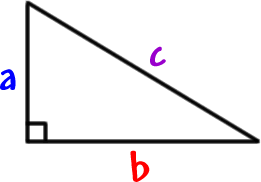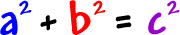Rectangle Info: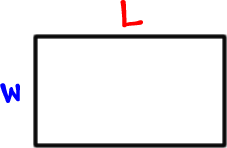areaperimeter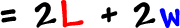Box info: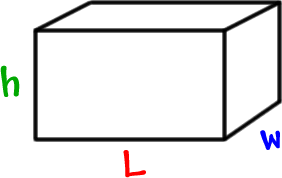volume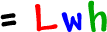surface area